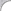## BWD Chapter 02: How stuff works

### 2004-10-25 16:24 - Beginners Web Development

Chapter 02
How stuff works

Computers are very fancy machines. It seems like they can do a million and one different things. From a wide perspective, at least. From a sufficiently narrow perspective, a computer can only do one thing: Examine a wire, and see if there is a voltage on it, or if there is not. Yes, the famous binary, one and zero. Everything in computers boils down to binary numbers, ones and zeros. The "magic" comes in when you take a whole lot of ones and zeros and put them together in special ways.

Here comes a lesson. Computers only do one and zero. Anything more complex and a computer can't handle it, on it's own. Of course, we don't leave computers to fend for themselves. We work all the time to fit more capability into our computers. The lesson is that a bunch of ones and zeros together can do wonderful things, but only when we pre-define what it means when we put all these ones and zeros together.

If you have ever shopped for computer memory, you probably heard about how many megabytes the memory could hold. What's a megabyte? It's a pre-defined concept. It's a particular number of bytes. In fact, it's 220 bytes. That's 2 to the power of 20. Don't get discouraged at the math, in chapter one we learned that computers are just big adding machines. If we want to get grimy with the computers insides, we need to be comfortable with math. But a megabyte is too big for now. For now, just know that a megabyte is a whole lot of bytes. A bit over a million bytes. So what's a byte? A byte is eight bits. So what's a bit? A bit is a binary digit. That's a one or a zero.

A binary digit, a bit, is the heart of computing. Bits are everything. A bit, being binary, differs in one special way from the digits that we are used to doing math with. It's binary, and our numbers are decimal. Look at the prefixes: bi- and deci-. Bi- means two, deci- means ten. A bicycle has two wheels, a decimal digit has ten possible values. Of course, they are: 0, 1, 2, 3, 4, 5, 6, 7, 8 and 9. A binary digit has two possible values: 0 and 1. Note that we always start at zero. This is important for the math, and important for computers. In computers, many things start at zero, even when a human mind might like to start counting at one.

So what can we do with two possible values? Everything we can do with ten! It just takes more digits. Look closely at the following math example:

```  1
+9
---
10```

Surely we all remember learning to carry the one in grade school? Well think about it a bit more closely. What happens when we add one to nine is we get ten. But, we only have ten digits, zero through nine, so we can't fit ten into the one digit that we have been using. We make a new column, and put the extra ten over there. With a one! Yes, our next column is the "tens column" and whatever we put in there, we really have ten of them. So, in decimal, "10" means "one ten, and no ones." The first column is the ones column, and each column left we go is worth ten times more.

Binary is the same way, except we have only two values. The first column is still the ones column. But we can only represent two values with it, zero and one. If we want to write two, we can't fit it in the one's column. What do we do? Make a new column. This time we're in binary, base two. The next column is the twos column.

```  1
+1
---
10```

Yes, one plus one is 10. In binary, the second column is the twos column, and in binary 10 means "one two and no ones." Or, two. Here's our first exersize in the series. Binary addition. If I've explained it well, and you've read it all and made sure you understood it as you went along this should be easy. We're also introducing our answer format. All answers are black text on a black background. Viewed in the original medium, my web page, at least. Select the text with your mouse and it will become white-on-blue and you can read it. Don't cheat, you'll only be cheating yourself! Each exersize should come with a longer answer explanation as well, and sometimes a hint, which will be labeled, so be sure to check for them.

```  11001
+10011
-------
101100```

Answer: The answer, as shown above, is 101100. How can we check our answer? Convert to decimal. The first number, 11001, means: 1